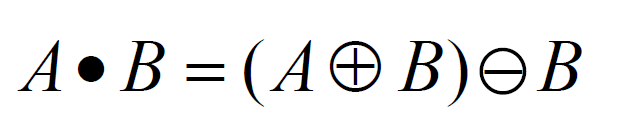# Python | Morphological Operations in Image Processing (Closing) | Set-2

In the previous article, the Opening operator was specified which was applying the erosion operation after dilation. It helps in removing the internal noise in the image. Closing is similar to the opening operation. In closing operation, the basic premise is that the closing is opening performed in reverse. It is defined simply as a dilation followed by an erosion using the same structuring element used in the opening operation.Syntax: cv2.morphologyEx(image, cv2.MORPH_CLOSE, kernel)

Parameters:
-> image: Input Image array.
-> cv2.MORPH_CLOSE: Applying the Morphological Closing operation.
-> kernel: Structuring element.

Below is the Python code explaining Closing Morphological Operation –

 `# Python programe to illustrate ` `# Closing morphological operation ` `# on an image ` ` `  `# organizing imports   ` `import` `cv2   ` `import` `numpy as np   ` ` `  `# return video from the first webcam on your computer.   ` `screenRead ``=` `cv2.VideoCapture(``0``) ` ` `  `# loop runs if capturing has been initialized. ` `while``(``1``): ` `    ``# reads frames from a camera ` `    ``_, image ``=` `screenRead.read() ` `     `  `    ``# Converts to HSV color space, OCV reads colors as BGR  ` `    ``# frame is converted to hsv ` `    ``hsv ``=` `cv2.cvtColor(image, cv2.COLOR_BGR2HSV) ` `     `  `    ``# defining the range of masking ` `    ``blue1 ``=` `np.array([``110``, ``50``, ``50``]) ` `    ``blue2 ``=` `np.array([``130``, ``255``, ``255``]) ` `     `  `    ``# initializing the mask to be ` `    ``# convoluted over input image ` `    ``mask ``=` `cv2.inRange(hsv, blue1, blue2) ` ` `  `    ``# passing the bitwise_and over ` `    ``# each pixel convoluted ` `    ``res ``=` `cv2.bitwise_and(image, image, mask ``=` `mask) ` `     `  `    ``# defining the kernel i.e. Structuring element ` `    ``kernel ``=` `np.ones((``5``, ``5``), np.uint8) ` `     `  `    ``# defining the closing function  ` `    ``# over the image and structuring element ` `    ``closing ``=` `cv2.morphologyEx(mask, cv2.MORPH_OPEN, kernel) ` `    `  `    ``# The mask and closing operation ` `    ``# is shown in the window  ` `    ``cv2.imshow(``'Mask'``, mask) ` `    ``cv2.imshow(``'Closing'``, closing) ` `     `  `    ``# Wait for 'a' key to stop the program  ` `    ``if` `cv2.waitKey(``1``) & ``0xFF` `=``=` `ord``(``'a'``): ` `        ``break` ` `  `# De-allocate any associated memory usage   ` `cv2.destroyAllWindows() ` ` `  `# Close the window / Release webcam  ` `screenRead.release() `

My Personal Notes arrow_drop_upCheck out this Author's contributed articles.

If you like GeeksforGeeks and would like to contribute, you can also write an article using contribute.geeksforgeeks.org or mail your article to contribute@geeksforgeeks.org. See your article appearing on the GeeksforGeeks main page and help other Geeks.

Please Improve this article if you find anything incorrect by clicking on the "Improve Article" button below.

Article Tags :

Be the First to upvote.

Please write to us at contribute@geeksforgeeks.org to report any issue with the above content.